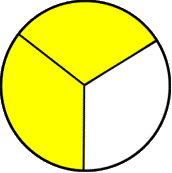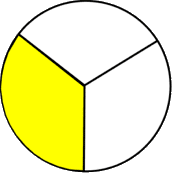1
visibility

Which of the given models shows a one-third?

• A• BThe correct answer is.

Please Note:

A fraction represents a part of a whole.

For instance:

• each part of a shape, when divided into two equal parts, is called a half.
• each part of a shape, when divided into three equal parts, is called a third.
• each part of a shape, when divided into four equal parts, is called a fourth.
• each part of a shape, when divided into five equal parts, is called a fifth.

Solution:

We consider all the fraction models one by one in order to identify the fraction showing a one-third.

• We consider the first fraction model.The given fraction model shows three equal parts.
The shaded part of the fraction model shows two-thirds.
Two-thirds means two of three equal parts.
• Looking at the second fraction model.The given fraction model shows three equal parts.
The shaded part of the fraction model shows a one-third.
One-third means one of three equal parts.

Therefore, the fraction modelshows a one-third.